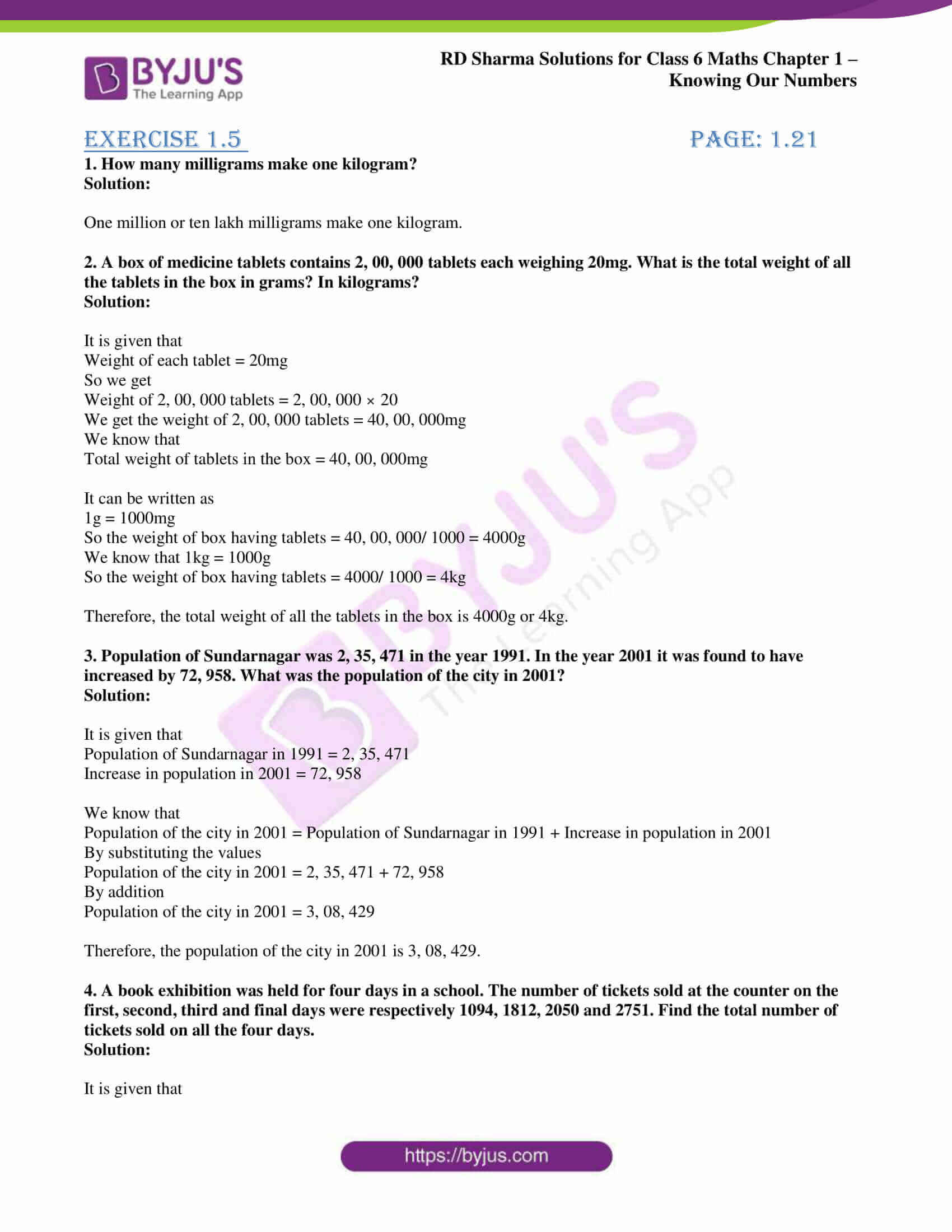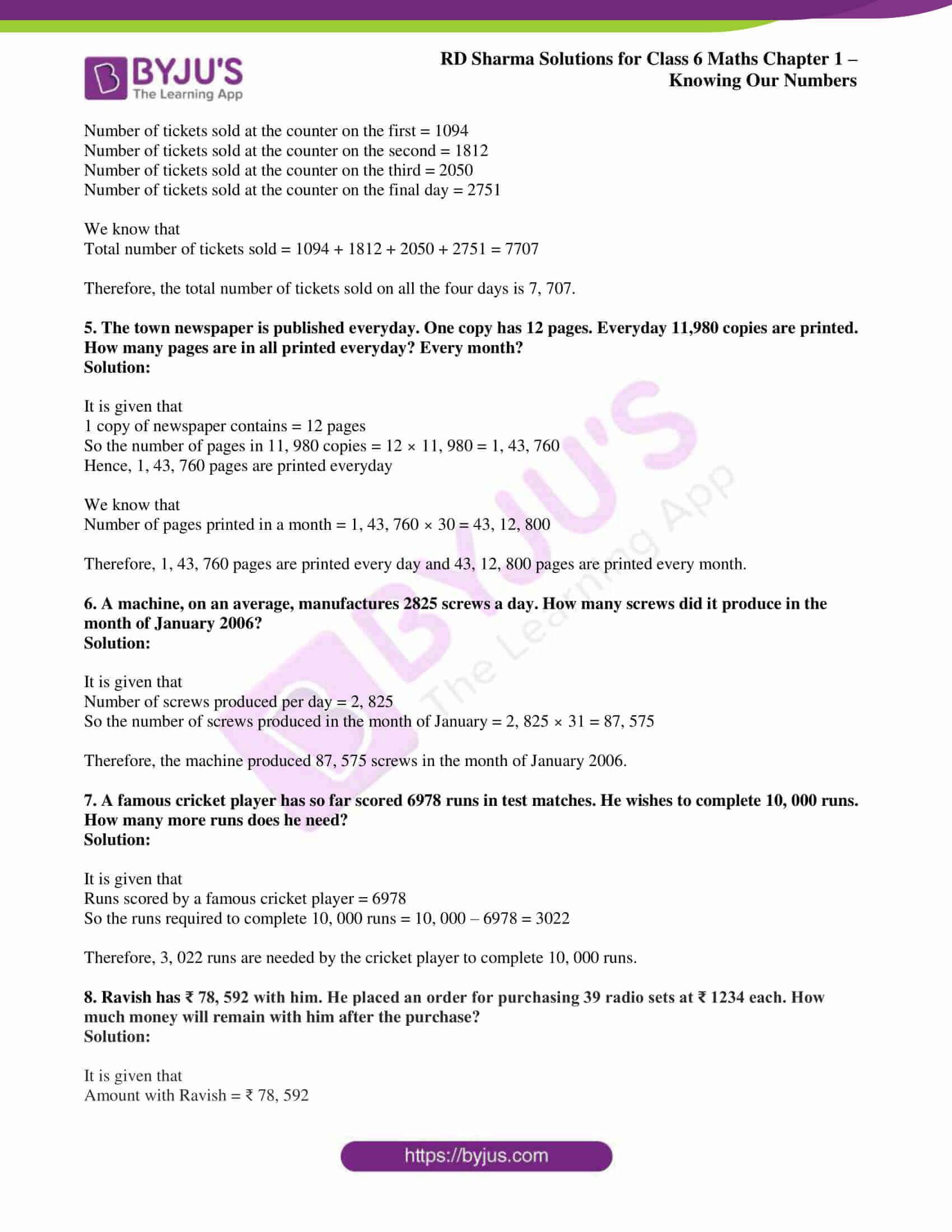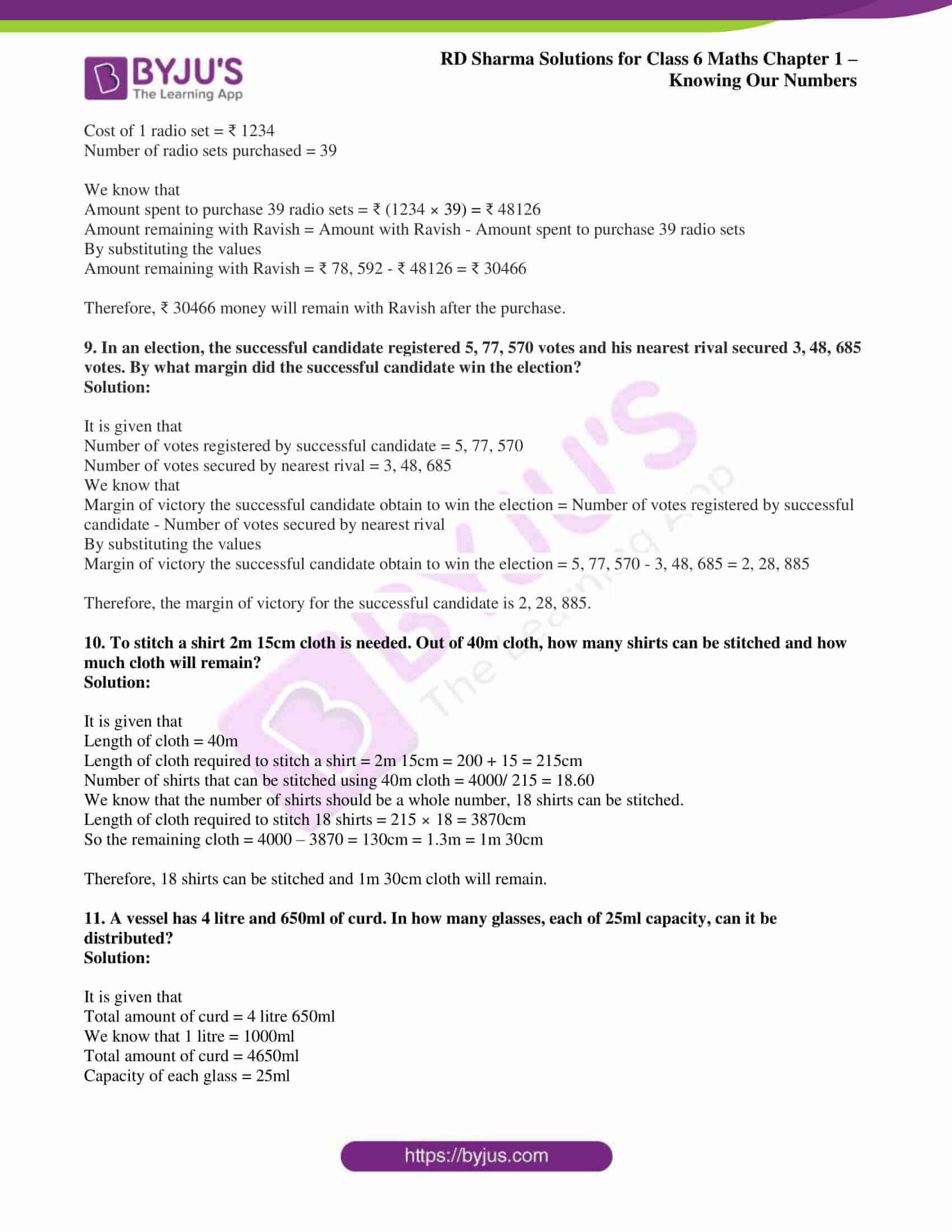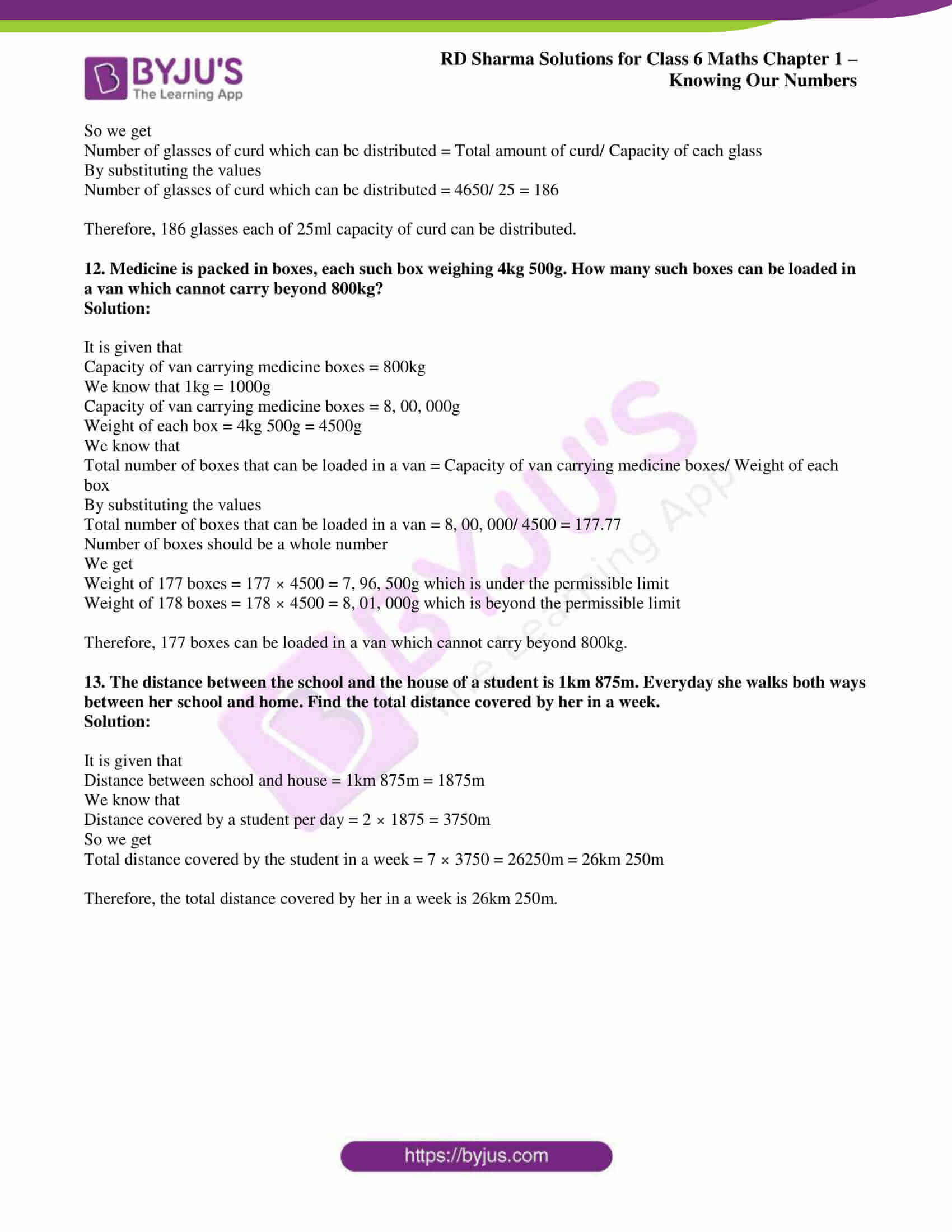# RD Sharma Solutions for Class 6 Maths Chapter 1: Knowing Our Numbers Exercise 1.5

## RD Sharma Solutions for Class 6 Maths Exercise 1.5 PDF

RD Sharma Solutions help students obtain conceptual knowledge and a better score in the exam. The solutions are created by teachers at BYJU’S having vast experience in the education industry. The PDF is made easily accessible for the students in order to help them solve exercise wise problems with a better understanding of concepts covered. Each solution contains explanations in an interactive manner in a simple language to make it easy for the students to understand. RD Sharma Solutions for Class 6 Maths Chapter 1 Knowing Our Numbers Exercise 1.5 are provided here.

## RD Sharma Solutions for Class 6 Maths Chapter 1: Knowing Our Numbers Exercise 1.5 Download PDF## Access answers to Maths RD Sharma Solutions for Class 6 Chapter 1: Knowing Our Numbers Exercise 1.5

#### Exercise 1.5 page: 1.21

1. How many milligrams make one kilogram?

Solution:

One million or ten lakh milligrams make one kilogram.

2. A box of medicine tablets contains 2, 00, 000 tablets each weighing 20mg. What is the total weight of all the tablets in the box in grams? In kilograms?

Solution:

It is given that

Weight of each tablet = 20mg

So we get

Weight of 2, 00, 000 tablets = 2, 00, 000 × 20

We get the weight of 2, 00, 000 tablets = 40, 00, 000mg

We know that

Total weight of tablets in the box = 40, 00, 000mg

It can be written as

1g = 1000mg

So the weight of box having tablets = 40, 00, 000/ 1000 = 4000g

We know that 1kg = 1000g

So the weight of box having tablets = 4000/ 1000 = 4kg

Therefore, the total weight of all the tablets in the box is 4000g or 4kg.

3. Population of Sundarnagar was 2, 35, 471 in the year 1991. In the year 2001 it was found to have increased by 72, 958. What was the population of the city in 2001?

Solution:

It is given that

Population of Sundarnagar in 1991 = 2, 35, 471

Increase in population in 2001 = 72, 958

We know that

Population of the city in 2001 = Population of Sundarnagar in 1991 + Increase in population in 2001

By substituting the values

Population of the city in 2001 = 2, 35, 471 + 72, 958

Population of the city in 2001 = 3, 08, 429

Therefore, the population of the city in 2001 is 3, 08, 429.

4. A book exhibition was held for four days in a school. The number of tickets sold at the counter on the first, second, third and final days were respectively 1094, 1812, 2050 and 2751. Find the total number of tickets sold on all the four days.

Solution:

It is given that

Number of tickets sold at the counter on the first = 1094

Number of tickets sold at the counter on the second = 1812

Number of tickets sold at the counter on the third = 2050

Number of tickets sold at the counter on the final day = 2751

We know that

Total number of tickets sold = 1094 + 1812 + 2050 + 2751 = 7707

Therefore, the total number of tickets sold on all the four days is 7, 707.

5. The town newspaper is published everyday. One copy has 12 pages. Everyday 11,980 copies are printed. How many pages are in all printed everyday? Every month?

Solution:

It is given that

1 copy of newspaper contains = 12 pages

So the number of pages in 11, 980 copies = 12 × 11, 980 = 1, 43, 760

Hence, 1, 43, 760 pages are printed everyday

We know that

Number of pages printed in a month = 1, 43, 760 × 30 = 43, 12, 800

Therefore, 1, 43, 760 pages are printed every day and 43, 12, 800 pages are printed every month.

6. A machine, on an average, manufactures 2825 screws a day. How many screws did it produce in the month of January 2006?

Solution:

It is given that

Number of screws produced per day = 2, 825

So the number of screws produced in the month of January = 2, 825 × 31 = 87, 575

Therefore, the machine produced 87, 575 screws in the month of January 2006.

7. A famous cricket player has so far scored 6978 runs in test matches. He wishes to complete 10, 000 runs. How many more runs does he need?

Solution:

It is given that

Runs scored by a famous cricket player = 6978

So the runs required to complete 10, 000 runs = 10, 000 – 6978 = 3022

Therefore, 3, 022 runs are needed by the cricket player to complete 10, 000 runs.

8. Ravish has ₹ 78, 592 with him. He placed an order for purchasing 39 radio sets at ₹ 1234 each. How much money will remain with him after the purchase?

Solution:

It is given that

Amount with Ravish = ₹ 78, 592

Cost of 1 radio set = ₹ 1234

Number of radio sets purchased = 39

We know that

Amount spent to purchase 39 radio sets = ₹ (1234 × 39) = ₹ 48126

Amount remaining with Ravish = Amount with Ravish – Amount spent to purchase 39 radio sets

By substituting the values

Amount remaining with Ravish = ₹ 78, 592 – ₹ 48126 = ₹ 30466

Therefore, ₹ 30466 money will remain with Ravish after the purchase.

9. In an election, the successful candidate registered 5, 77, 570 votes and his nearest rival secured 3, 48, 685 votes. By what margin did the successful candidate win the election?

Solution:

It is given that

Number of votes registered by successful candidate = 5, 77, 570

Number of votes secured by nearest rival = 3, 48, 685

We know that

Margin of victory the successful candidate obtain to win the election = Number of votes registered by successful candidate – Number of votes secured by nearest rival

By substituting the values

Margin of victory the successful candidate obtain to win the election = 5, 77, 570 – 3, 48, 685 = 2, 28, 885

Therefore, the margin of victory for the successful candidate is 2, 28, 885.

10. To stitch a shirt 2m 15cm cloth is needed. Out of 40m cloth, how many shirts can be stitched and how much cloth will remain?

Solution:

It is given that

Length of cloth = 40m

Length of cloth required to stitch a shirt = 2m 15cm = 200 + 15 = 215cm

Number of shirts that can be stitched using 40m cloth = 4000/ 215 = 18.60

We know that the number of shirts should be a whole number, 18 shirts can be stitched.

Length of cloth required to stitch 18 shirts = 215 × 18 = 3870cm

So the remaining cloth = 4000 – 3870 = 130cm = 1.3m = 1m 30cm

Therefore, 18 shirts can be stitched and 1m 30cm cloth will remain.

11. A vessel has 4 litre and 650ml of curd. In how many glasses, each of 25ml capacity, can it be distributed?

Solution:

It is given that

Total amount of curd = 4 litre 650ml

We know that 1 litre = 1000ml

Total amount of curd = 4650ml

Capacity of each glass = 25ml

So we get

Number of glasses of curd which can be distributed = Total amount of curd/ Capacity of each glass

By substituting the values

Number of glasses of curd which can be distributed = 4650/ 25 = 186

Therefore, 186 glasses each of 25ml capacity of curd can be distributed.

12. Medicine is packed in boxes, each such box weighing 4kg 500g. How many such boxes can be loaded in a van which cannot carry beyond 800kg?

Solution:

It is given that

Capacity of van carrying medicine boxes = 800kg

We know that 1kg = 1000g

Capacity of van carrying medicine boxes = 8, 00, 000g

Weight of each box = 4kg 500g = 4500g

We know that

Total number of boxes that can be loaded in a van = Capacity of van carrying medicine boxes/ Weight of each box

By substituting the values

Total number of boxes that can be loaded in a van = 8, 00, 000/ 4500 = 177.77

Number of boxes should be a whole number

We get

Weight of 177 boxes = 177 × 4500 = 7, 96, 500g which is under the permissible limit

Weight of 178 boxes = 178 × 4500 = 8, 01, 000g which is beyond the permissible limit

Therefore, 177 boxes can be loaded in a van which cannot carry beyond 800kg.

13. The distance between the school and the house of a student is 1km 875m. Everyday she walks both ways between her school and home. Find the total distance covered by her in a week.

Solution:

It is given that

Distance between school and house = 1km 875m = 1875m

We know that

Distance covered by a student per day = 2 × 1875 = 3750m

So we get

Total distance covered by the student in a week = 7 × 3750 = 26250m = 26km 250m

Therefore, the total distance covered by her in a week is 26km 250m.

### RD Sharma Solutions Class 6 Maths Chapter 1 – Knowing Our Numbers Exercise 1.5

RD Sharma Solutions Class 6 Maths Chapter 1 Knowing Our Numbers Exercise 1.5 explains the conversion of numbers from kilometer to meter, meter to centimeter, centimeter to millimeter and so on.

### Key features of RD Sharma Solutions for Class 6 Maths Chapter 1: Knowing Our Numbers Exercise 1.5

• The solutions are well structured by the experts based on latest CBSE guidelines and exam pattern.
• Important formulas and conversions are highlighted and pictorial representation is given for better understanding among students.
• The solutions are available in PDF format which can be downloaded effortlessly by students.
• The expert team at BYJU’S create solutions with the aim of improving knowledge among students.